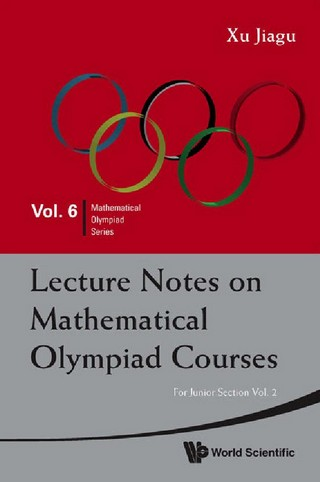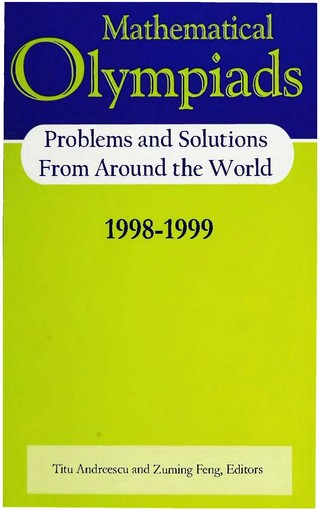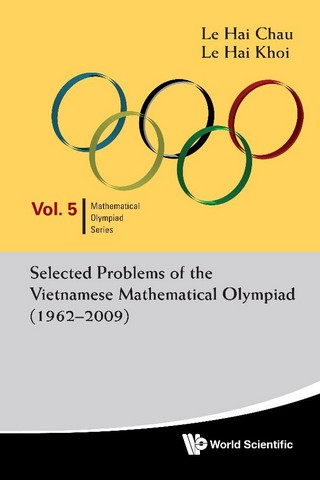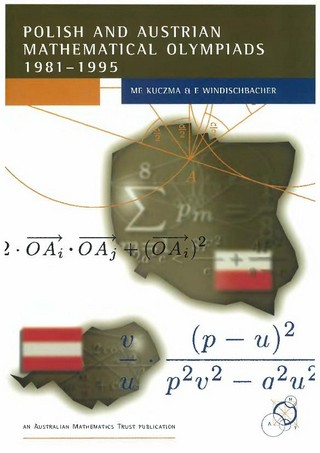### Book Toankho

Sách hay - Sách đẹp

Browsing:

## USA and International Mathematical Olympiads 2004The Mathematical Olympiad examinations, covering the USA Mathematical Olympiad (USAMO) and the International Mathematical Olypiad (IMO), have been published annually since 1976. The IMO is the world mathematics championship for high school students. It takes place every year in a Read more…

## Lecture Notes on Mathematical Olympiad Courses (For Junior Section, Vol. 2)Olympiad mathematics is not a collection of techniques of solving mathematical problems but a system for advancing mathematical education. This book is based on the lecture notes of the mathematical Olympiad training courses conducted by the author in Singapore. Its Read more…

## Lecture Notes on Mathematical Olympiad Courses (For Junior Section, Vol. 1)Olympiad mathematics is not a collection of techniques of solving mathematical problems but a system for advancing mathematical education. This book is based on the lecture notes of the mathematical Olympiad training courses conducted by the author in Singapore. Its Read more…

## USA and International Mathematical Olympiads 2006-2007## USA and International Mathematical Olympiads 2003The Mathematical Olympiad examinations, covering the USA Mathematical Olympiad (USAMO) and the International Mathematical Olympiad (IMO), have been published annually since 1976. This is the fourth volume in that series. The IMO is a world mathematics competition for high school Read more…

## USA and International Mathematical Olympiads 2007-2008## Mathematical Olympiads (Problems and Solutions From Around the World 1998-1999)This volume contains a large range of problems, with and without solutions, taken from 25 national and regional mathematics olympiads from around the world, and the problems are drawn from several years’ contests. In many cases, more than one solution Read more…

## Selected Problems of the Vietnamese Mathematical Olympiad (1962 – 2009)Vietnam has actively organized the National Competition in Mathematics and since 1962, the Vietnamese Mathematical Olympiad (VMO). On the global stage, Vietnam has also competed in the International Mathematical Olympiad (IMO) since 1974 and constantly emerged as one of the Read more…